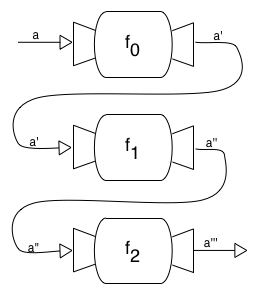# Left-to-right function composition

Function composition is a way of combining two functions to produce a new function. If we have a function $f :: b \to c$ and a function $g :: a \to b$, we can combine $f$ and $g$ to produce a new function $f \circ g :: a \to c$. In Haskell this is defined as f . g = \x -> f (g x). So “f compose g” means call g with an argument, and pass the result as the argument to f. We can use this to assemble chains of multiple functions such as a . b . c . ….

Composed functions are read right-to-left; f = a . b . c is equivalent to f x = a (b (c x)). So if we have a composed function (++ "!") . (++ " world") . reverse and call it with “olleh”, it will first be reversed to give “hello”, then have “world” appended, then “!” to give us “hello world!”.

I recently had a case where I wanted to compose functions, but I was thinking about the problem in a slightly different way. Rather than composing right-to-left, I wanted to perform a series of operations, and chain the output from the first function into the input of the second function, and so on.The output of each function is passed as input to the next

In other words, I wanted left-to-right composition, rather than right-to-left. To do this we can define a new operator to reverse the order in which functions are composed.

It turns out that Haskell already has this operator defined in Control.Arrow, so all I really needed was import Control.Arrow.

As an aside, I also realised that Haskell lets us use line-breaks and indents if we want to write either form of composition in a more imperative style, similar to do-notation: